Robotic Systems and Applications

Published: 31 December 2022

# Research of the dynamical properties of mechatronic prosthesis

Justas Tamošiūnas1
Vytautas Bučinskas2
1, 2Department of Mechatronics, Robotics and Digital Manufacturing, Vilnius Tech, Vilnius, Lithuania
Corresponding Author:
Justas Tamošiūnas
Views 20

#### Abstract

The mechatronic ankle prosthesis plays a crucial role in the recreation of natural gait biomechanics by being able to actively control time-torque parameters in different sub-phases of the walking cycle. This paper presents a methodology for improving the design process of the individual characteristics of the object of interest. A series of tests were taken to derive a correlation between an actual structure and a developed mathematical model to determine the parameters of the object under investigation. The model provides a possibility to determine time-changing force-related properties to capture a full picture of the structure for which a particular design is being chosen. The method also acts as a tool to expand traditional design criteria to get the overall view of the structural dynamics of the mechanical system.

## 1. Introduction

Prostheses for below-knee amputees are typically passively constructed mechanical structures with a major disadvantage to reproduce all the characteristics of the biological gait cycle – various studies have shown that the lack of ability to actively generate energy during a specific walking cycle period results in both general fatigue and possible health issues -. To address these problems, active prostheses for below-knee amputees were developed and are electrically controlled mechanical structures which are mostly orientated to reflect a typical walking gait where both kinematics and dynamics are taken as characteristics to be assumed -. The electrically active prostheses were initially developed to provide a positioning of the structure which was the most important aim for the active control – these types of structures showed a capability to set the structure position to the gait starting state , . Since then, several various actively controlled prostheses were introduced mainly showing active-energy characteristics only for kinematics of the structure but not for the additive force generation that a biological limb is capable of , . Types of control were related to resistance change, constrainment of elasticity, shift of the moment arm, etc. As technology developed the iBiom angle joint was introduced by H. Herr showing the efficiency of active system involvement in the overall gait and not only in the positioning as it was , , . Controlled dynamics of the structure were seen both in kinematic characteristics (amplitudes of the movement, ability to change position in terms of gait patterns, etc.) and dynamics – the rate at which all preferable movement is reached . Although the new development of active control implementation to the overall walking cycle was seen as a high improvement, the consequence of reducing the kinematic capabilities was introduced due to the complexity and the nature of the movement in the investigated joint , . A need for complex design decisions reduced the level of a structure to meet its initial goal – to help regain the lost movement of the amputee. The kinematic capabilities of a prosthesis depend on various variables and design goals. The stability, strength and resistance of a structure, reliability, cost-effectiveness and design consistency are the factors that demand a simple structure while the increase in the level of natural movement reflection introduces the unpreferable complexity which is reflected in the degrees of freedom in the system , , .

Environmental conditions for a structure are also variables for the end-kinematics to be seen: level of activeness, a need for mobility, and the intensity of the disease can also affect the decision in design to be made.

All of these different variables act as additional complexity parameters thus introducing time-consuming, cost-inefficient and additional error factors -. Most of these are addressed in traditionally based calculation methods which not only shift the initial goal for a prosthetic to mimic the behaviour of a biological limb but also do not provide intuitively understood dynamics as are based on the static evaluation. Dynamic parameter evaluation demands a need for evaluation of a change rate in parameters to be seen. Time-domain change is a parameter from itself as a quotative value. The rate at which the parameter of the structure is changing can be misleading in terms of the value of the rate excluded from the overall picture. Actively controlled prostheses are highly change-rate dependent structures that do not base their initial behaviour only on static value characteristics , . These time-dependent parameters are based on the environmental characteristics which are derived from the typical walking gait cycle , . All time-dependent characteristics are the result which is seen as the rate of angular displacement over certain periods which are indicated by different phases of the structure's working cycle itself. These variable-based phases are differentiated into foot-on-ground and foot-off-ground types. The phase of interest in designing an actively controlled structure is the foot-on-ground phase with its own five sub-phases to be used as qualitative end-start areas with a particular displacement rate to be evaluated . The structurally most intensive sub-phase of the foot-on-ground gait cycle phase is the additional force where the object of investigation should provide the highest amount of energy in the shortest period – the energy to time ratio is in its highest value , , . Without a clear indication of whether the provided energy for this particular sub-phase is sufficient enough to reach a desired angular displacement after a certain time, major design flaws can be seen such as delayed energy provision, lack of work exceeded by the prosthetic and the overall disturbance of the gait cycle leading not on only to inefficient walking experience but also to probable additional health issued due to unsymmetric muscle work , . All of these time-based energy solutions should be addressed in every degree of freedom to evaluate the effect of complex movement and the probability of additional energy losses both based on amplitude and change rate terms. A particular method for describing the needed rate-change parameters of the structure is related to the mathematical modelling techniques which describe the output of the actively affected system. The system chosen for a tool to be later used is validated with a physical model and later compared to the predictable dynamics of the structure with the derived tool.

The main aim of this work was to create a model for an actively controlled system evaluation in terms of dynamically changing characteristics of the structure under investigation. The resultant time domain parameters of the model should work as an indicative tool to design a structure including not only strength based but also rate change decisions. Model input parameters such as mass, torque, moment arm, etc. allow choosing qualitative design parameters for an individual structure as well as forecasting the probable dynamic behaviour of the structure of the investigation.

Results have shown a considerably high correlation ratio in terms of amplitude response for both force and displacement values, therefore a strong statement can be placed for further development research in the area of interest.

As there is no common practice to use mathematical models of such kind in the development of personalized prosthetics in the field of the gait rehabilitation process, the presented model has the potential to be used as a standardized tool for custom solutions of the actively controlled prosthetics.

This paper is composed of five general sections: Introduction, Modelling, Experiments, Results and discussion, and Conclusion. Modelling section covers methodology and simulation subsections. Experiments section is composed of theoretical background, methodology and error calculation subsections. Results and discussion section comprises modelling results, experimental results and comparison subsections.

## 2. Modelling

### 2.1. Methodology

The method of analysis implemented is orientated to the analytical-mathematical description of the object of interest. A derived dedicated system can evaluate individual structural design parameters based on the initial conditions which are typically related to the gait cycle characteristics. The basic idea behind the derived mathematical model is an ability to calculate amplitudes, velocities and accelerations of the movement from different boundary conditions mostly affected by individual needs of the movement to be restored.

For the aforementioned implementation of the dedicated system derivation, a standard methodology of describing a movement is used. Mass-spring-damper system is derived to replicate a movement of the mechanical relations in the object of interest by covering the basic principles of motion. The derived model is later used to derive an equation of motion based on the second Newton’s law.

These equations are integrated into the computational software to have an iterative tool for needed kinematics calculations. The general mechanical equation used:

1
$\left[m\right]\left\{\stackrel{¨}{x}\right\}+\left[h\right]\left\{\stackrel{˙}{x}\right\}+\left[k\right]\left\{x\right\}=\left\{F\left(t\right)\right\}.$

### 2.2. Model of mechanical system

The object of interest is comprised form the basic structural elements: a gear from two lead screws, two belt gears, two motors, an elastic element and other rotational joints. For simplicity of the mathematical model derivation, a symmetrical modelling approach is used to derive a system representing half of all the components as all stiffness, damping and mass parameters are used to cover the overall structural elements and the behaviour of interest.

Fig. 1The modelled object of interest – a mechatronic ankle prosthesis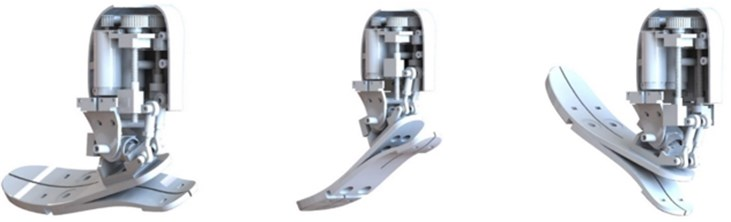All-important structural parts of the modelled mechatronic prosthesis are replicated with dedicated mass for each part while the stiffness and damping effects are represented with individual springs and dampers for stiffness and dampening. All the dedicated parameters for each element are given in Table 1. The general idea behind the mass-spring model covers the activation of the motor by transferring rotational movement through the belt gears to the pulleys which are rigidly connected to the lead screw. The later movement of the lead nut is described as the movement with dampening due to the inertia of the motor’s rotor. The movement from the lead nut is then transferred to the deformation of the flexible foot as the additional constrainment comes from the parallel spring. The affected foot element through the rotational joint of the ankle is transferring movement to the ground.

Equations of motion were derived for both vertical and horizontal movement as given below. The general equations for vertical movement are:

2
${m}_{4}{\stackrel{¨}{x}}_{4}+{k}_{3}{x}_{4}+{b}_{1}{\stackrel{˙}{x}}_{4}-{k}_{3}{x}_{5}=F,$
3
${m}_{5}{\stackrel{¨}{x}}_{5}+{k}_{3}{x}_{5}+{k}_{4}{x}_{5}+{k}_{5}{x}_{5}+{k}_{6}{x}_{5}-{k}_{3}{x}_{4}=0.$

The general equations for horizontal movement are:

4
$\left({m}_{2}+{m}_{3}\right){\stackrel{¨}{x}}_{\frac{2}{3}}+{k}_{1}{x}_{\frac{2}{3}}+{k}_{2}{x}_{\frac{2}{3}}-{k}_{2}{x}_{4}=F,$
5
${m}_{4}{\stackrel{¨}{x}}_{4}+{k}_{2}{x}_{4}-{k}_{2}{x}_{\frac{2}{3}}=0.$

Fig. 2Mass-spring-damper system of the modelled mechatronic ankle prosthesis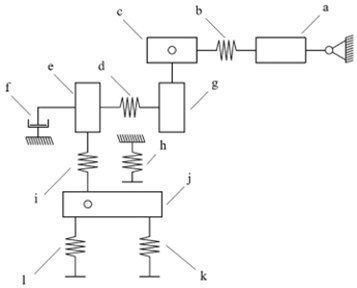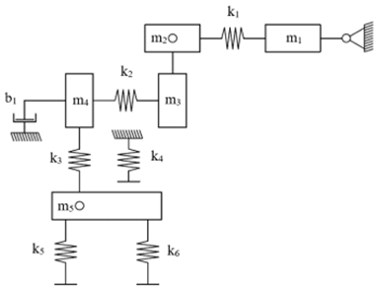Table 1Parameters and indication of components used for mass-spring-damper system

 Component Description Indication Value Units a Motor ${m}_{1}$ 0.325 kg b Motor-belt joint stiffness ${k}_{1}$ 244 N/m c Belt-gear pulley ${m}_{2}$ 0.034 kg d Lead screw-nut stiffness ${k}_{2}$ 1500 N/m e Lead-screw nut ${m}_{4}$ 0.12 kg f The inertia of the rotor ${b}_{1}$ 0.31 Ns/m g Lead-screw ${m}_{3}$ 0.094 kg h Spring stiffness ${k}_{4}$ 330 N/m i Stiffness of the end-foot ${k}_{3}$ 1200 N/m j Stiffness of the front-foot ${m}_{5}$ 0.542 kg k Stiffness of the bottom foot ${k}_{6}$ 1200 N/m l Stiffness of the heel ${k}_{5}$ 1200 N/m

## 3. Simulation

Simulation of the representative mass-spring-damper systems is implemented with the Matlab Simulink software in which all the parts of the modelled object of interest are represented by the dedicated mathematical block which in turn represents the equation of motion.

The derived equations for the computational model for the vertical movement are:

6
${\stackrel{¨}{x}}_{4}=\frac{1}{{m}_{4}}\left(F-{k}_{3}{x}_{4}-{b}_{1}{\stackrel{˙}{x}}_{4}+{k}_{3}{x}_{5}\right),$
7
${\stackrel{¨}{x}}_{5}=\frac{1}{{m}_{5}}\left(-{k}_{3}{x}_{5}-{k}_{4}{x}_{5}-{k}_{5}{x}_{5}-{k}_{6}{x}_{5}+{k}_{3}{x}_{4}\right).$

The derived equations for the computational model for the horizontal movement are:

8
${\stackrel{¨}{x}}_{2/3}=\frac{1}{{m}_{2}+{m}_{3}}\left(F-{k}_{1}{x}_{\frac{2}{3}}-{k}_{2}{x}_{\frac{2}{3}}+{k}_{2}{x}_{4}\right),$
9
${\stackrel{¨}{x}}_{4}=\frac{1}{{m}_{4}}\left(-{k}_{2}{x}_{4}+{k}_{2}{x}_{\frac{2}{3}}\right).$

Fig. 3Matlab Simulink model for movement in the vertical and horizontal directions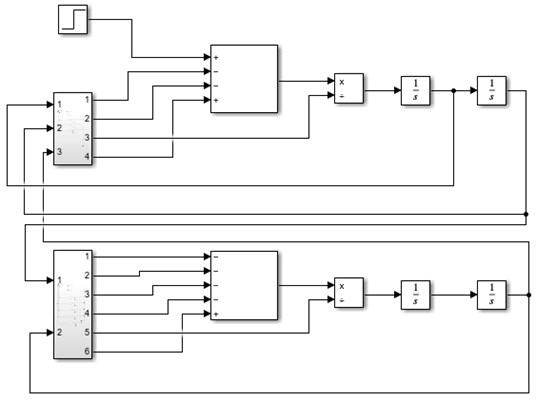## 4. Experiments

### 4.1. Theoretical background

For the experimental part of the research, a built model of the modelled ankle prosthesis is used. The object under investigation is designed and constructed in such a way that all the main structural contributors to the overall dynamics of the model would be represented in the derived mathematical model also. As the overall gait cycle is comprised of several walking phases and sub-phases, the most demanding in terms of actively controlled movement is chosen for the comparison – the additional force sub-phase – plantarflexion – is chosen as the work done by the prosthesis at this sub-phase is not only in highest demand but also it is the core property of the actively controlled prosthetics. Design and test-related activities are generally orientated to the aforementioned motions and analysis of it.

### 4.2. Methodology

For the test of the generated force of the object of interest a force plate, BTS P-6000 is used. The dedicated reaction force measurements were taken for the plantarflexion motion as the measured force is later compared with the calculated acceleration of the foot mass from the mathematical model. For the ankle joint angular movement (both displacement and velocity) two approaches are implemented – angular displacement measurement with MP6050 gyroscopes and using potentiometers placed on every rotational joint of the structure of the investigation. All measurements were taken with three configurations: at the neutral position (N) (ankle rotation in the frontal plane is 0 deg), at the highest inversion position (IN) (ankle rotation in the frontal plane is –15 deg) and at the highest eversion position (EV) (ankle rotation in the frontal plane is 15 deg). There were 10 samples taken for each plantarflexion movement in each configuration as mentioned before. All general technical specifications are given in Table 2.

Table 2Technical specification of the used instruments

 BTS P-600 MP6U050 Potentiometer Sampling frequency 500 Hz / 1000 Hz 500 Hz 10 kHz Range –8000 N – 8000 N 360 deg 300 deg Resolution 0.12 N (16 bit) 0.35 deg (10 bit) 0.29 deg (10 bit) Deviation 2 % 1.75 deg 1.45 deg

Fig. 4The object of interest on the BTS P-6000 force plate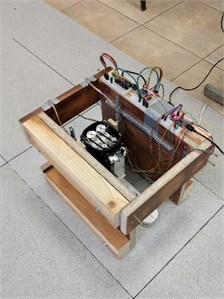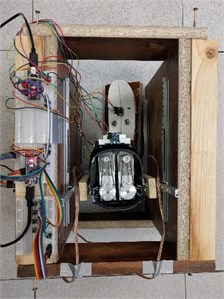Fig. 5The object of interest with MPU6050 and potentiometers sensors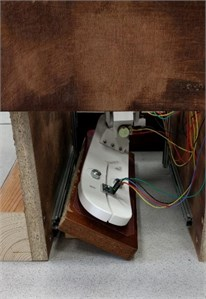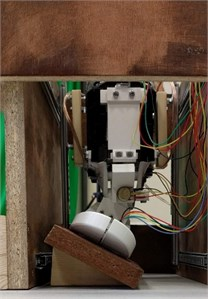### 4.3. Error calculation

All relates statistical calculations in terms of taken tests were made by calculating standard deviation and variance. A relative error calculation was used to determine the differences between the results for tests and simulations with the derived mathematical model.

Standard deviation was calculated by:

10
$\sigma =\sqrt{\frac{\sum {\left({x}_{i}-\mu \right)}^{2}}{N}}.$

The variance was calculated by:

11
${S}^{2}=\frac{\sum \left({x}_{i}-\stackrel{-}{x}{\right)}^{2}}{n-1}.$

Relative error was calculated by:

12
$\delta =\left|\frac{{\upsilon }_{A}-{\upsilon }_{E}}{{\upsilon }_{E}}\right|\cdot 100\mathrm{%}.$

## 5. Results and discussion

### 5.1. Modelling results

As initial outputs of the model are related to the kinematics of the movement – linear displacement, velocity and acceleration – and additional post-processing is needed to get the results for the comparison with the experimental outcomes. A time-history result of changing force, angular displacement and angular velocity are needed. To calculate the force in the time domain, an acceleration change and mass of the moving object are taken. For the angular velocity – linear velocity is taken and the radius of the object of interest is used to calculate the angular change rate. For the angular displacement – all calculated linear displacement values are used to calculate the angular displacement of the moving object with a particular radius. Values for all the calculations are given in Table 3.

Table 3Results from the mathematical model

 Ac. Units Force Units Vel. Units a.Vel. Units Disp. Units a.Dis Units AP 1.20 m/s2 2.10 N 0.17 m/s 32.0 deg/s 0.13 m 38.0 deg ST 0.20 s 0.20 s 0.20 s 0.20 s 0.11 s 32.0 s AP.T. 0.05 s 0.05 s 0.05 s 0.05 s 0.20 s 0.20 s AP.R. N/A N/A N/A N/A N/A N/A N/A N/A 0.05 s 0.05 s

### 5.2. Experiment results

Experimental results were taken as raw values of force in newtons (BTS P-6000 force plate), and angular displacement in degrees (MP6050 post-processed in the Arduino IDE to get the angular displacement), angular displacement in units of potentiometer voltage change (correlated to be equal to 0.525 degrees). Angular velocity of the joint was calculated by differentiating angular displacement. Potentiometer values of movement in the frontal plane were used to determine the stability of the joint during plantarflexion of the object of interest. All values for calculated results from the experimental test are given in Tables 4, Table 5 and Table 6.

Table 4Results from the tests with the force plate

 Force (N) Units Force (EV) Units Force (IN) units AMP 1.80 N 1.60 N 1.30 N ST 0.40 s 0.50 s 0.52 s AMP. T. 0.20 s 0.02 s 0.06 s

Table 5Results from the tests with the accelerometer

 a. Disp. (N) Units a. Disp. (EV) Units a. Disp. (IN) Units AMP 42.0 deg 31.0 deg 32.0 deg AMP. R. 38.0 deg 29.0 deg 31.0 deg ST 0.06 s 0.04 s 0.04 s AMP. T. 0.02 s 0.02 s 0.02 s

Table 6Results from the tests with the potentiometer

 a. Disp. (N) Units a. Disp. (EV) Units a. Disp. (IN) Units AMP 40.95 deg 34.13 deg 32.03 deg AMP. R. 4.95 deg 34.13 deg 32.03 deg ST 0.25 s 0.30 s 0.22 s AMP. T. 0.25 s 0.30 s 0.22 s

### 5.3. Comparison

Comparison is given in form of plots for both experimental results and outputs of the mathematical model. For every given plot a dedicated table indicates the error and difference for amplitude value, response time and time to reach the amplitude value. For every test, standard deviation and variance values are also shown to inspect the validity of experimental results.

### 5.3.1. Force comparison

Fig. 6Comparison plots between simulated and test results, acceleration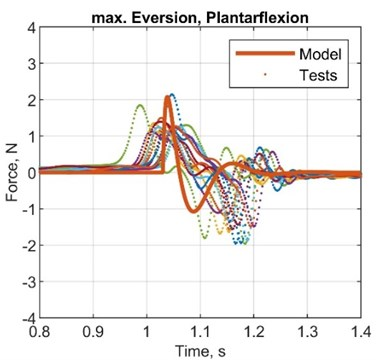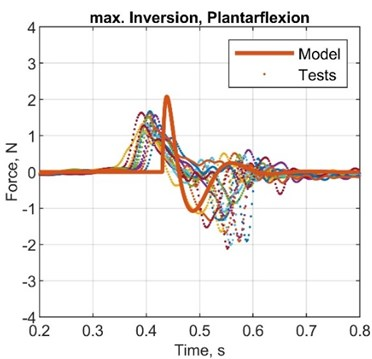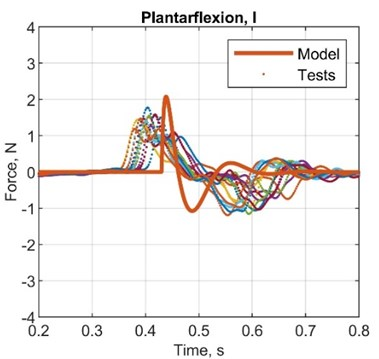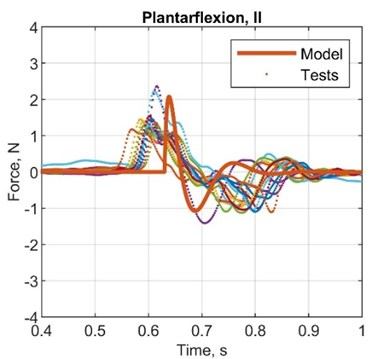Table 7Comparison between simulated and test results, acceleration

 1st plot 2nd plot 3rd plot 4th plot AMP diff., N 0.30 0.50 0.50 0.80 ST diff., s –0.20 –0.25 -0.30 –0.32 AMP. T diff., s –0.15 –0.05 0.03 –0.01 AMP error, % 16.7 31.3 31.3 61.5 ST error, % –50.1 –55.6 –60.1 –61.1 AMP. T error, % –75.1 –50.4 150.2 –16.5 STD, N 0.27 0.32 0.32 0.35 VAR, N2 0.62 0.84 0.89 1.03

### 5.3.2. Angular displacement comparison (MPU6050)

Fig. 7Comparison plots between simulated and test results, angular displacement (MPU6050)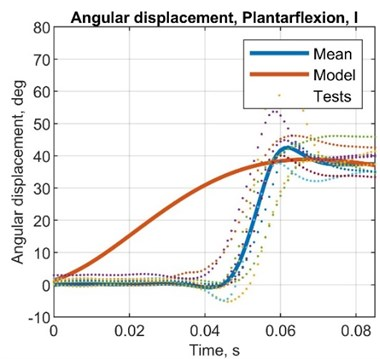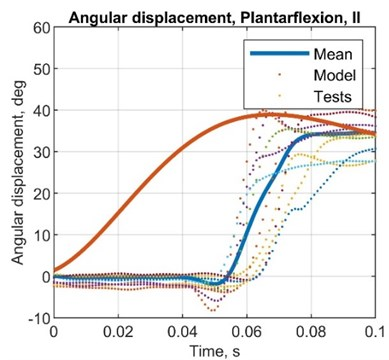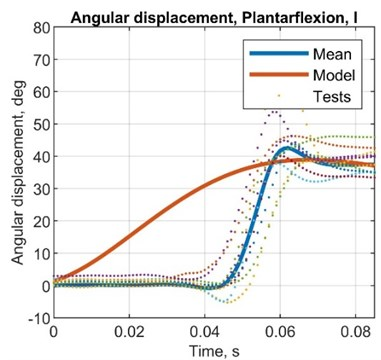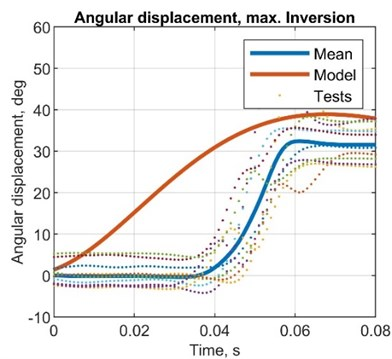Table 8Comparison between simulated and test results, angular displacement

 1st plot 2nd plot 3rd plot 4th plot AMP diff., deg –4.00 3.00 7.00 6.00 AMP R. diff, deg –6.00 –2.00 3.00 1.00 ST diff, s 0.14 0.17 0.16 0.16 AMP T. diff, s 0.03 0.02 0.03 0.03 AMP error, % –9.52 8.57 22.6 18.8 AMP R. error, % –15.8 –5.88 10.3 3.23 ST error, % 233.3 566.7 395.5 499.1 AMP T. error, % 150.2 66.7 149.1 148.3 STD, deg 2.61 3.48 2.62 3.19 VAR, deg2 0.07 0.12 0.07 0.10

### 5.3.3. Angular displacement comparison (potentiometer)

Fig. 8Comparison plots between simulated and test results, angular displacement (potentiometer)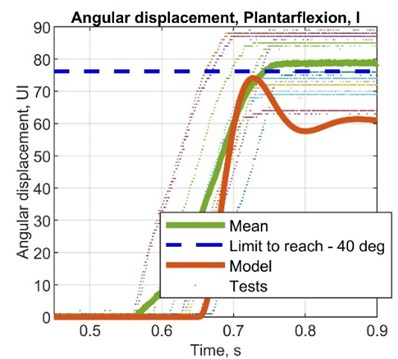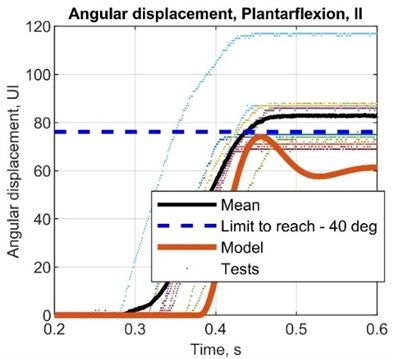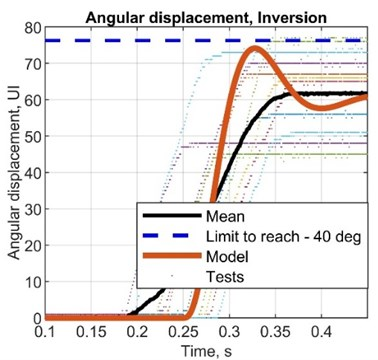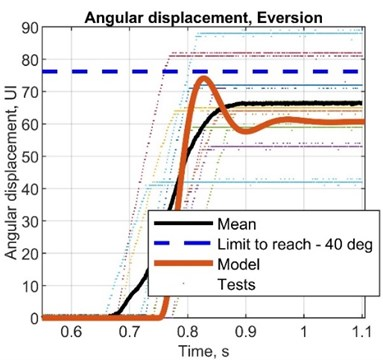Table 9Simulated values for displacement and calculated for angular displacement

 1st plot 2nd plot 3rd plot 4th plot AMP diff., deg –3.00 –13.1 10.4 14.1 AMP R. diff, deg –16.1 –26.5 –3.32 1.12 ST diff, s –0.05 –0.05 –0.10 –0.02 AMP T. diff, s –0.20 –0.20 –0.25 –0.17 AMP error, % –3.85 –14.8 15.3 22.9 AMP R. error, % –20.5 –29.6 –4.62 1.64 ST error, % –20.4 –20.8 –33.3 –9.09 AMP T. error, % –80.2 –80.6 –83.5 –77.2 STD, deg 6.86 7.65 5.52 5.89 VAR, deg2 2.38 3.00 1.57 1.80

### 5.3.4. Angular velocity comparison

Fig. 9Comparison plots between simulated and test results, angular velocity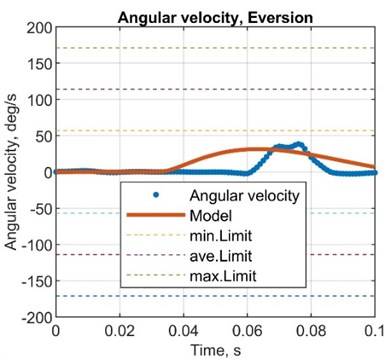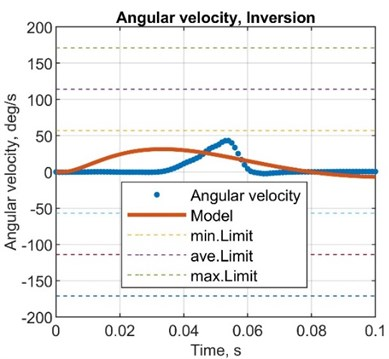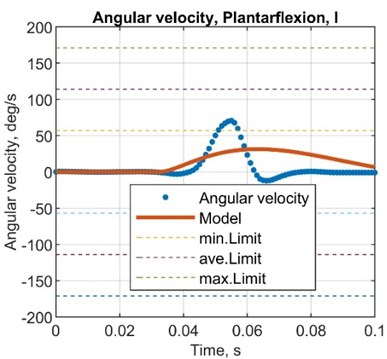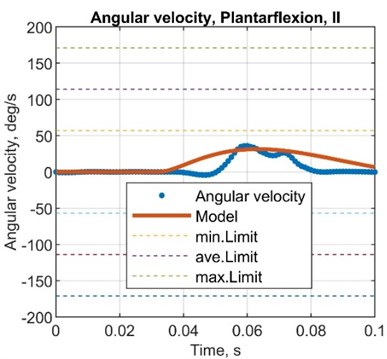Table 10Comparison between simulated and test results, angular velocity

 1st plot 2nd plot 3rd plot 4th plot AMP diff., deg/s –30.2 –6.12 –8.15 –16.1 ST diff., s 0.14 0.15 0.16 0.15 AMP. T diff., s 0.02 0.02 0.04 0.03 AMP error, % –48.4 –15.8 –20.1 –33.3 ST error, % 233.3 295.1 388.1 295.4 AMP. T error, % 42.9 66.7 233.3 149.2

## 6. Conclusions

Here established mathematical model is suitable to determine the characteristics of the dynamic behaviour of the object of interest – the mechatronic system of the actively controlled prosthesis. Simulated results showed the same pattern behaviour in terms of both amplitude and response time. Experiments were conducted for three different configurations for the most demanding sub-phase of the system (plantarflexion) covering nominal, highest amplitude inversion and eversion positions. Three parameters – angular displacement, angular velocity and angular acceleration – were compared by calculating differences and errors for amplitude, response time and time to reach the favourable value of the parameter under interest. Comparison of acceleration of the joint was conducted through exerted force values as the error at the plantarflexion reached 24.1 %, –52.9 % and –62.8 % for the amplitude, response time and time to reach the highest amplitude, respectively. Corresponding errors at the inversion and eversion positions showed values of 46.4 %, 60.6 % and –16.7 %. Angular displacement error values for amplitude, reached displacement, response time and time to reach the amplitude have shown –9.1 %, –10.8 %, 482.1 % and 180.5 %, respectively. Corresponding error values for positions in inversion and eversion reached 20.7 %, 6.8 %, 447.3 % and 148.7 %. Values from the potentiometer showed errors of –9.3 %, –25.1 %, –20.6 % and –80.4 %. Angular speed comparison has indicated the error of –32.1 %, 264.2 % and 54.8 % as for positions in inversion and eversion –26.7 %, 341.8 % and 191.3 % were reached. In respect of the calculated error values, the amplitude response seems to have the closest representative behaviour as the response time in terms of angular displacement and velocity does not indicate a high correlation ratio. The overall patterns of the exerted force seem to replicate the calculated one by the mathematical model as the simulated response of the angular displacement and angular velocity are a good indicator only in terms of the amplitude value.